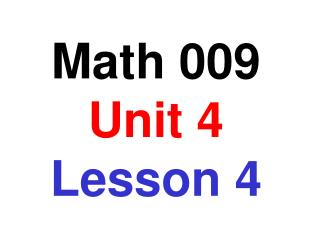DownloadDownload PresentationMath 009 Unit 4 Lesson 4

# Math 009 Unit 4 Lesson 4

Download Presentation## Math 009 Unit 4 Lesson 4

- - - - - - - - - - - - - - - - - - - - - - - - - - - E N D - - - - - - - - - - - - - - - - - - - - - - - - - - -
##### Presentation Transcript

1. Math 009 Unit 4Lesson 4

2. By definition Ö25 is the number you would multiply times itself to get 25 for an answer. Because we are familiar with multiplication, we know that Ö25 = 5 Numbers like 25, which have whole numbers for their square roots, are called perfect squares You need to memorize at least the first 15 perfect squares

3. Square root Square root Perfect square Perfect square 1 81 Ö1 = 1 Ö81 = 9 4 100 Ö4 = 2 Ö100 = 10 9 121 Ö9 = 3 Ö121 = 11 16 144 Ö16 = 4 Ö144 = 12 25 169 Ö25 = 5 Ö169 = 13 36 196 Ö36 = 6 Ö196 = 14 49 225 Ö49 = 7 Ö225 = 15 64 Ö64 = 8

4. Every whole number has a square root Most numbers are not perfect squares, and so their square roots are not whole numbers. Most numbers that are not perfect squares have square roots that are irrational numbers Irrational numbers can be represented by decimals that do not terminate and do not repeat The decimal approximations of whole numbers can be determined using a calculator

5. Obj: To find the square root of a number • Find the square roots of the given numbers • If the number is not a perfect square, use a calculator to find the answer correct to the nearest thousandth. 81 Ö81 = 9 37 Ö37 » 6.083 158 Ö158 » 12.570

6. Obj: To find the square root of a number • Find two consecutive whole numbers that the given square root is between • Try to do this without using the table Ö16 = 4 and Ö25 = 5 so Ö18 Ö18 is between 4 and 5 Ö115 Ö100 = 10 and Ö121 = 11 so Ö115 is between 10 and 11

7. Multiplying radicals The product of the square roots of two numbers is the same as the square root of the product of the numbers Examples: Ö3 · = Ö12 Ö36 Ö7 · = Ö11 Ö77

8. Simplify the following expressions -Ö4 = -2 = 7 8 + 9 · 7Ö64 + 9 = 56 + 9 = 65 5 + = 5 5 + 7 · Ö25 Ö49 = 25 + 7 = 32

9. Simplify the following expressions Ö 4 Ö4 2 = = 81 Ö81 9 1 1 Ö 1 Ö 1 – – = 12 6 36 144 1 2 – = 12 12 1 = 12

10. Simplified radical form No factor inside the radical should be a perfect square. Ö18 = = Ö2 = 3 Ö2 Ö9 2 Ö9 · Ö108 = = Ö3 = 6 Ö3 Ö36 3 Ö36 · Ö96 = = Ö6 = 4 Ö6 Ö16 6 Ö16 ·

11. Graphing real numbers The graph of a number is a dot placed where the number would be on the number line 12 Graph the number: 3 -10 -5 0 5 10 Graph the number: -8.5 -10 -5 0 5 10

12. Graphing inequalities The symbol, [ , means that the point is included in the graph The symbol, ( , means that the point is not included in the graph Graph the real numbers between -4 and 6 ( ) -10 -5 0 5 10 Graph the real numbers less than 8 ) -10 -5 0 5 10

13. Graphing inequalities The symbol, [ , means that the point is included in the graph The symbol, ( , means that the point is not included in the graph Graph the inequality: x > -3 ( -10 -5 0 5 10 Graph the inequality: x £4 ] -10 -5 0 5 10